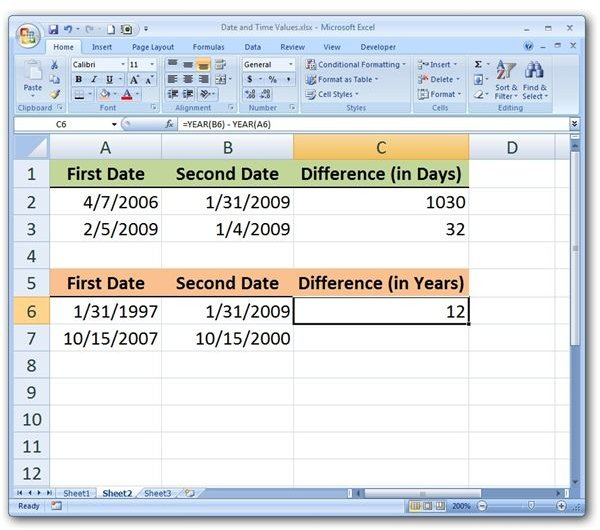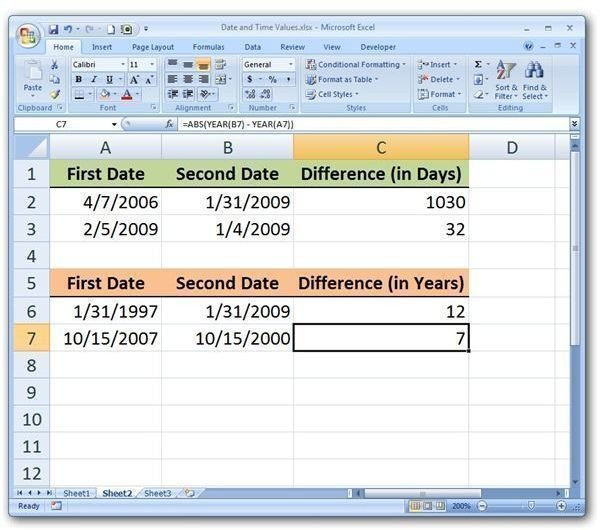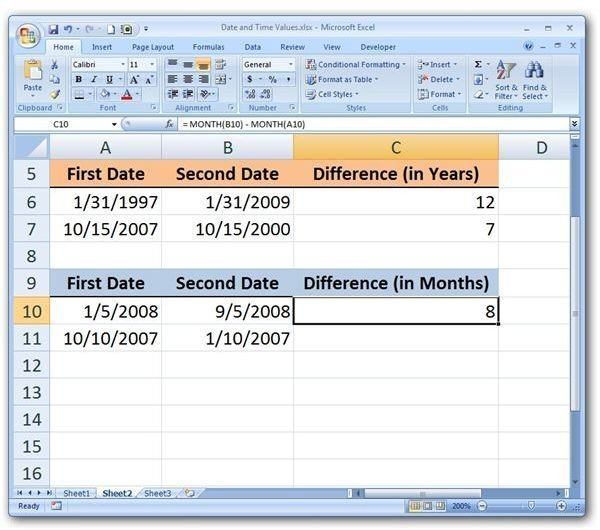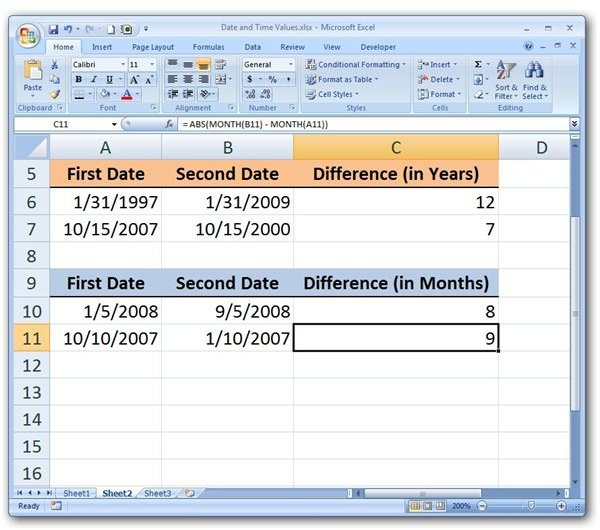# How to Subtract Date Values in Microsoft Excel

Page content

## Different Ways to Subtract Dates

There are several types of computations that involve dates and subtraction in Excel. For instance, you may want to find the difference in days, months, or years between two dates in order to determine the amount of time that passed from the beginning to the end of an event. Similarly, if given only a single date, you may need to determine an exact date in the past that occurred a specific number of days ago.

For the most part, these calculations are fairly straightforward in Excel, but there are a few tips and tricks that you can use to make the resulting answers easier to obtain and more useful. This is especially true if you want to convert your answers from a raw number of days to months and years.

## Finding the Number of Days between Two Dates

If you’re only interested in determining the number of days between two dates, the calculation is very simple. All you have to do is subtract the two cells. That is, if the first date is in cell A2 and the second one in cell B2, you can use the basic formula

= B2 – A2

as shown in the screenshot below. (Click any image for a larger view.)

If you’re trying to construct a basic formula that you can use for an entire list of date calculations, you might want to embellish this just a bit. In long lists of data, it’s possible that the larger of two dates in any row might reside in either column, and the basic formula above could end up giving a negative answer for some of these rows.

If you want to make sure that the number of days is always positive, you can modify this formula with the ABS function. This function will return the absolute value of any difference between the two dates so you won’t have to worry about negative numbers appearing in your results. The modified formula would appear as

= ABS(B3 – A3)

and an example of this is shown below.## Finding the Number of Years between Two Dates

There are times when the number of days between two dates isn’t quite the information we need. Instead, we’d prefer to know the number of years. In this case, we can utilize Excel’s YEAR function in the calculation. A basic example of the use of this function is the following.

= YEAR(B6) – YEAR(A6)

This is also shown in the following screenshot.Just as in the previous section, we can incorporate the ABS function into this formula to ensure that the result is always positive. This would yield the formula:

= ABS(YEAR(B7) - YEAR(A7))## Finding the Number of Months between Two Dates

Calculating the number of months between two dates within the same year is analogous to calculating the number of years between two dates. The only difference is that the MONTH function is used instead of the YEAR function. This is shown in the following example.

= MONTH(B10) - MONTH(A10)Or, if we want to make sure that the result isn’t a negative number, we can include the ABS function.

= ABS(MONTH(B11) - MONTH(A11))There is a more precise way of calculating the number of years between two dates that will take into account partial years. This method can also be used to calculate the number of months between two dates with more precision, whether they are in the same year or not. We discuss this more in the tutorial on the YEARFRAC function.

Interested in other Excel tutorials? Be sure to check out the other user guides available at Bright Hub, including our collection of Microsoft Excel function tutorials.